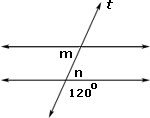Definition Of Alternate Interior Angles

When two lines are cut by a transversal, the pair of angles formed interior of the two lines and on the opposite of the transversal is called Alternate Interior Angles.

If two parallel lines are cut by a transversal, the alternate interior angles are congruent

Examples of Alternate Interior Angles

In the figure shown, l is the transversal that cut the pair of lines. Angles a and d and angles b and are alternate interior angles.Solved Example on Alternate Interior Angles

Ques: Find the measure of angle m in the figure shown.Choices:

A. 120o
B. 180o
C. 60o
D. Insufficient information